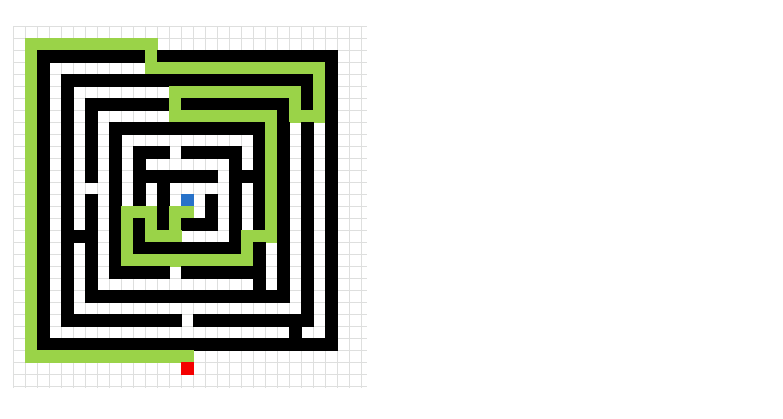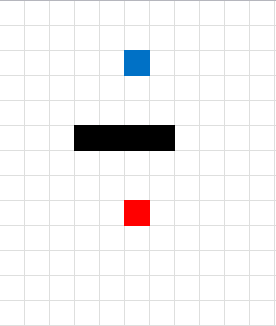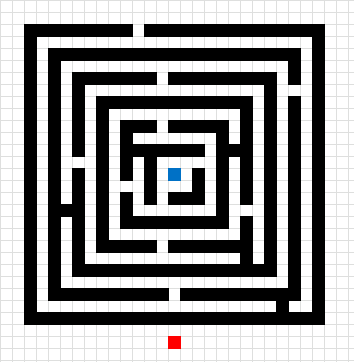Author: Oscar Cronquist Article last updated on February 16, 2021Two months ago I posted some interesting stuff I found: Shortest path. Let me explain, someone created a workbook that calculated the shortest path between a start cell and an end cell in a maze, and it did that using only formulas. Pretty amazing. The formulas were made from Dijkstra's Algorithm. I tried to create a larger maze but the workbook grew too large.

I then found the A * pathfinding algorithm and it is a lot easier for the computer to calculate. There is a great explanation of the A * pathfinding algorithm here: Introduction to A* Pathfinding.

Basically, there is an open list, a closed list and a final path. The open list contains cells that are being considered to find the final path. The closed list contains cells that we don't need to consider again.

They all contain coordinates or cells based on criteria. The criteria are:

1. The distance to the END cell. (The distance to the END cell is calculated with the Manhattan distance method.
2. The distance traveled from the start cell.

If the END cell is added to the open list, the closed list is finished calculating. Now it is time to find the final path. The final path is also found using the Manhattan distance method but it can only travel on cells in the closed list.

Here is an animated picture, it shows you how the macro works, simplified.

• The blue cell is the start cell
• The red cell is the end cell
• Gray cells are cells in the closed list.
• Green cells are the final path.
• Black cells are walls.Here is a slightly more advanced "map".This is a maze. I have removed the closed list cells to make the macro quicker.### VBA Code

```'Name macro
Sub FindShortestPath1()

'Disable screen refresh
Application.ScreenUpdating = False

'Dimension variables and their data types
Dim G() As Variant
Dim H() As Variant
Dim N() As Variant
Dim OL() As Variant
Dim CL() As Variant
Dim S() As Variant
Dim E() As Variant
Dim W() As Variant
Dim Gv() As Variant
Dim i As Single

'Redimension variables
ReDim S(0 To 1)
ReDim E(0 To 1)
ReDim W(0 To 3, 0 To 1)
ReDim OL(0 To 1, 0)
ReDim CL(0 To 1, 0)
ReDim Gv(0 To 3)

'Save values in cell range specified in named range "Area" to variable Rng
Rng = Range("Area").Value

'Save the upper limit of rows from Rng variable to variable a
a = UBound(Rng, 1) - 1

'Save the upper limit of columns from Rng variable to variable a
b = UBound(Rng, 2) - 1

'Redimension variables G, H and N based on variable a and b
ReDim G(0 To a, 0 To b)
ReDim H(0 To a, 0 To b)
ReDim N(0 To a, 0 To b)

'For ... next loop from 1 to the number of rows in Rng variable
For R = 1 To UBound(Rng, 1)

'For ... next loop from 1 to the number of columns in Rng variable
For C = 1 To UBound(Rng, 2)

'Check if cell Rng(r, C) is equal to "S" (start point)
If Rng(R, C) = "S" Then
'Save number in variable R - 1 to variable S position 0 (zero)
S(0) = R - 1

'Save number in variable C - 1 to variable S position 1
S(1) = C - 1
End If

'Check if cell Rng(r, C) is equal to "E" (end point)
If Rng(R, C) = "E" Then
E(0) = R - 1
E(1) = C - 1
End If

'Check if S(0) is not equal to "" and E(0) is not equal to ""
If S(0) <> "" And E(0) <> "" Then Exit For
Next C
Next R

'Save number stored in S(0) to array variable CL (closed list) position 0,0
CL(0, 0) = S(0) 'row

'Save number stored in S(1) to array variable CL (closed list) position 1,0
CL(1, 0) = S(1) 'column

'Save -1  to array variable W position 0,0
W(0, 0) = -1

'Save 1 to array variable W position 1,0
W(1, 0) = 1

'Save 0 to array variable W position 2,0
W(2, 0) = 0

'Save 0 to array variable W position 3,0
W(3, 0) = 0

'Save 0 to array variable W position 0,1
W(0, 1) = 0

'Save 0 to array variable W position 1,1
W(1, 1) = 0

'Save -1 to array variable W position 2,1
W(2, 1) = -1

'Save 1 to array variable W position 3,1
W(3, 1) = 1

'Save boolean value False to variable Echk
Echk = False

'Keep iterating until Echk is True
Do Until Echk = True

'For ... next statement
For i = 0 To UBound(CL, 2)

'For ... next statement
For j = 0 To 3

'Save boolean value False to variable Echk
chk = False

'Add number in CL position 0, i and W position j, 0 and save total to tr
tr = CL(0, i) + W(j, 0)

'Add number in CL and W and save total to tc
tc = CL(1, i) + W(j, 1)

If tr < 0 Or tc < 0 Or tr > UBound(Rng, 1) Or tc > UBound(Rng, 2) Then
chk = True
Else

For k = UBound(CL, 2) To 0 Step -1
If tr = CL(0, k) And tc = CL(1, k) Then
chk = True
Exit For
End If
Next k

If Rng(tr + 1, tc + 1) = 1 Then chk = True

For k = UBound(OL, 2) To 0 Step -1
If tr = OL(0, k) And tc = OL(1, k) Then
chk = True

If G(CL(0, i), CL(1, i)) + 1 < G(tr, tc) Then
G(tr, tc) = G(CL(0, i), CL(1, i)) + 1
H(tr, tc) = Abs(tr - E(0)) + Abs(tc - E(1))
N(tr, tc) = G(tr, tc) + H(tr, tc)
End If
Exit For
End If
Next k

If chk = False Then
OL(0, UBound(OL, 2)) = tr
OL(1, UBound(OL, 2)) = tc
ReDim Preserve OL(UBound(OL, 1), UBound(OL, 2) + 1)
G(tr, tc) = G(CL(0, i), CL(1, i)) + 1
H(tr, tc) = Abs(tr - E(0)) + Abs(tc - E(1))
N(tr, tc) = G(tr, tc) + H(tr, tc)
If Rng(tr + 1, tc + 1) = "E" Then Echk = True
End If
End If
Next j
Next i

If Echk <> True Then

For i = LBound(OL, 2) To UBound(OL, 2)
If OL(0, i) <> "" Then
Nchk = N(OL(0, i), OL(1, i))
Exit For
End If
Next i

For i = LBound(OL, 2) To UBound(OL, 2)
If OL(1, i) <> "" Then
If N(OL(0, i), OL(1, i)) < Nchk And N(OL(0, i), OL(1, i)) <> "" Then
Nchk = N(OL(0, i), OL(1, i))
End If
End If
Next i

For i = LBound(OL, 2) To UBound(OL, 2)
If OL(0, i) <> "" Then
If N(OL(0, i), OL(1, i)) = Nchk Then
ReDim Preserve CL(UBound(CL, 1), UBound(CL, 2) + 1)
CL(0, UBound(CL, 2)) = OL(0, i)
OL(0, i) = ""
CL(1, UBound(CL, 2)) = OL(1, i)
OL(1, i) = ""
End If
End If
Next i
End If
Loop

tr = E(0)
tc = E(1)

Schk = False
Do Until Schk = True

For i = UBound(CL, 2) To 0 Step -1
If CL(0, i) = (tr + 1) And CL(1, i) = tc _
And (Rng(tr + 2, tc + 1) <> "W" _
And Rng(tr + 2, tc + 1) <> "1") _
Then Gv(0) = G(tr + 1, tc)
If CL(0, i) = tr And CL(1, i) = (tc + 1) _
And (Rng(tr + 1, tc + 2) <> "W" _
And Rng(tr + 1, tc + 2) <> "1") _
Then Gv(1) = G(tr, tc + 1)
If CL(0, i) = (tr - 1) And CL(1, i) = tc _
And (Rng(tr, tc + 1) <> "W" _
And Rng(tr, tc + 1) <> "1") _
Then Gv(2) = G(tr - 1, tc)
If CL(0, i) = tr And CL(1, i) = (tc - 1) _
And (Rng(tr + 1, tc) <> "W" _
And Rng(tr + 1, tc) <> "1") _
Then Gv(3) = G(tr, tc - 1)

For j = 0 To 3
If Gv(j) <> "" Then Nf = Gv(j)
Next j
For j = 0 To 3
If Gv(j) < Nf And Gv(j) <> "" Then Nf = Gv(j)
Next j
Next i
Application.ScreenUpdating = True
Select Case Nf
Case Gv(0)
tr = tr + 1
Range("Area").Cells(tr + 1, tc + 1) = "W"
Rng(tr + 1, tc + 1) = "W"
Case Gv(1)
tc = tc + 1
Range("Area").Cells(tr + 1, tc + 1) = "W"
Rng(tr + 1, tc + 1) = "W"
Case Gv(2)
tr = tr - 1
Range("Area").Cells(tr + 1, tc + 1) = "W"
Rng(tr + 1, tc + 1) = "W"
Case Gv(3)
tc = tc - 1
Range("Area").Cells(tr + 1, tc + 1) = "W"
Rng(tr + 1, tc + 1) = "W"
End Select

If Rng(tr + 2, tc + 1) = "S" _
Or Rng(tr + 1, tc + 2) = "S" _
Or Rng(tr, tc + 1) = "S" _
Or Rng(tr + 1, tc) = "S" Then Schk = True

Loop
Application.ScreenUpdating = True
End Sub
```

### Get the Excel fileFind-shortest-path.xlsm